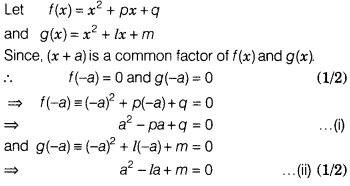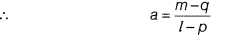# If(x + a) is a common factor of (x2 + px + q) and (x2 + lx + m), then find the value of a

If(x + a) is a common factor of (x2 + px + q) and (x2 + lx + m), then find the value of a.On subtracting Eq. (ii) from Eq. (i), we get
(l-p)a + q -m = 0
⇒ (l- p)a = m-q